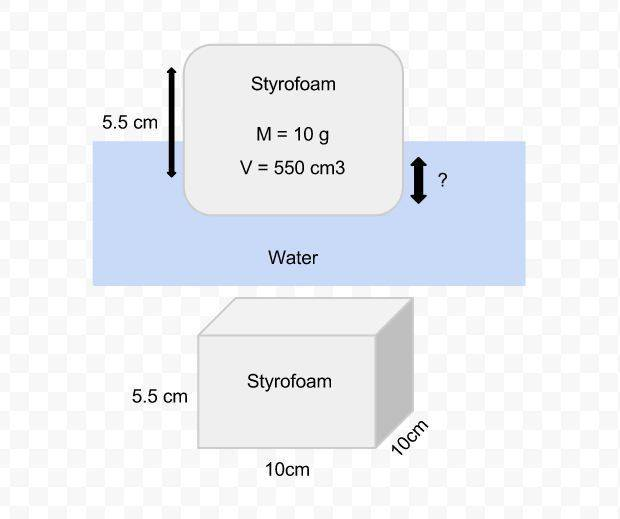# Amount of styrofoam submerged

I'm trying to find how much of a cube of styrofoam is submerged in water without measuring because the amount is very small.If I have a block of styrofoam with a mass of 10g and a density of 0.018g/cm3
How much of the styrofoam will be submerged?

MY guess would be 1mm, 10g of water has a volume of 10cm^3, so the same volume of styrofoam to displace same volume of water would be .1cm *10 cm * 10cm

I'm a little bit confused. Can you explain that again more thoroughly?

Any floating object displaces its own weight of fluid.

— Archimedes of Syracuse

So the weight of the styrofoam is 10g, to float, it must displace 10g of water

The density of water is 1g/cm^3 so 10g of water has a volume of 10cm^3. That is the volume of water to be displaced by an equal volume of styrofoam, since the block has a 100cm^2 bottom, it will take a height of 1mm to reach the desired volume.

Wow, simple. thanks!